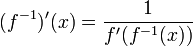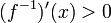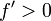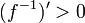Inverse of increasing function is increasing

Statement

Suppose$f$ is an increasing function on its domain. Then,$f$ is a one-one function and the inverse function$f^{-1}$ is also an increasing function on its domain (which equals the range of$f$).

Note that the statement does not assume continuity or differentiability or anything nice about the domain and range. In fact, the domain and range need not even be subsets of the reals. All we need is that they be totally ordered sets so that the notion of increasing makes sense.

Proof

Compatibility with inverse function theorem

This is not a proof but provides an illustration of why the statement is compatible with the inverse function theorem. In particular, the inverse function theorem can be used to furnish a proof of the statement for differentiable functions, with a little massaging to handle the issue of zero derivatives.

The inverse function theorem states that:$\! (f^{-1})'(x) = \frac{1}{f'(f^{-1}(x))}$

In particular, this tells us that if$\! f'(f^{-1}(x)) > 0$, then$(f^{-1})'(x) > 0$. In particular, if$f' > 0$ everywhere,$(f^{-1})' > 0$ everywhere.